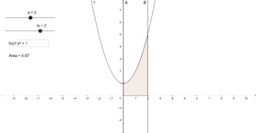•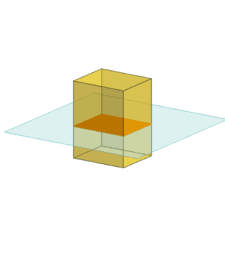Cross Sections & Nets

Book

•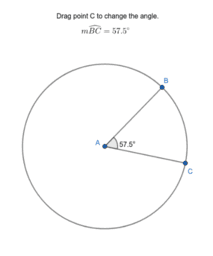Angles & Circles

Activity

•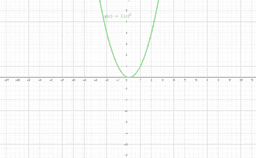Transformations of Graphs

Activity

•Activity

•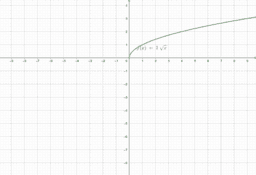Activity

•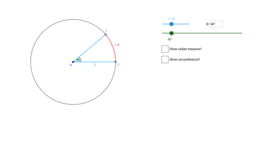Activity

•Activity

•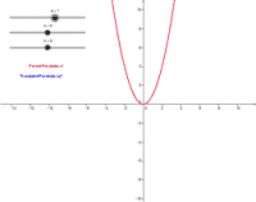Book

•Translating a Graph of a Quadratic Equation

Activity

•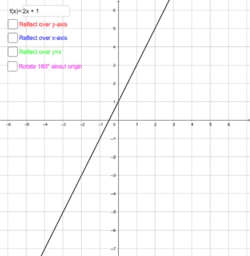Exploring Symmetry

Activity

•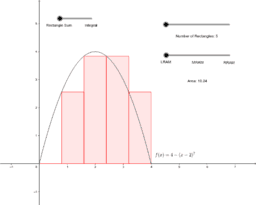Rectangular Sum Approximation & the Integral

Activity

•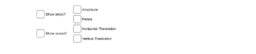Translations of the Graphs of Sine and Cosine

Activity

•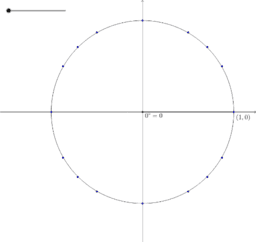Interactive Unit Circle

Activity

•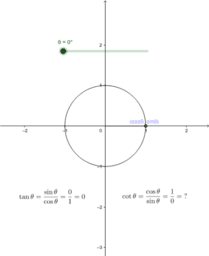Graphing Tangent and Cotangent

Activity

•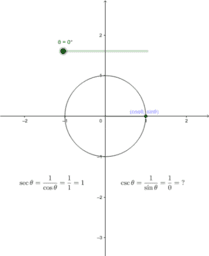Graphing Secant and Cosecant

Activity

•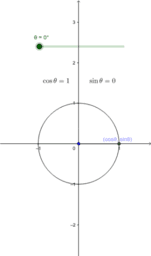Graphing Sine and Cosine

Activity

•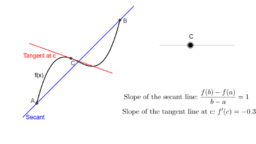Mean Value Theorem

Activity

•Volume of a Solid - Washer Method

Activity

•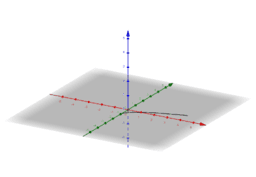Volume of a Solid - Disc Method

Activity

•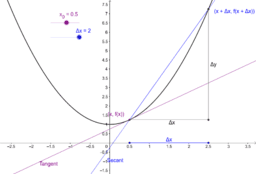Secant & Tangent Line

Activity

•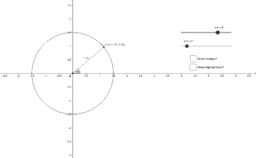Trigonometry on the Coordinate Plane

Activity

•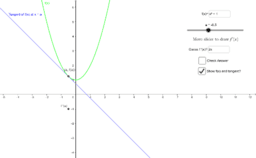Graphing the Derivative

Activity

•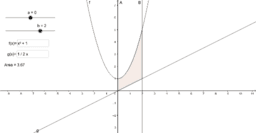Area Between Two Functions

Activity

•